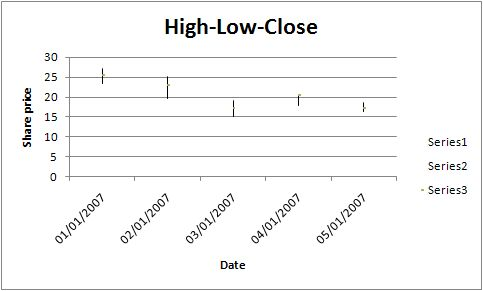and 1 contributors

# NAME

Stock - A class for writing Excel Stock charts.

# SYNOPSIS

To create a simple Excel file with a Stock chart using Excel::Writer::XLSX:

``````    #!/usr/bin/perl -w

use strict;
use Excel::Writer::XLSX;

my \$workbook  = Excel::Writer::XLSX->new( 'chart.xlsx' );

my \$chart = \$workbook->add_chart( type => 'stock' );

# Add a series for each High-Low-Close.
categories => '=Sheet1!\$A\$2:\$A\$6',
values     => '=Sheet1!\$B\$2:\$B\$6'
);

categories => '=Sheet1!\$A\$2:\$A\$6',
values     => '=Sheet1!\$C\$2:\$C\$6'
);

categories => '=Sheet1!\$A\$2:\$A\$6',
values     => '=Sheet1!\$D\$2:\$D\$6'
);

# Add the worksheet data the chart refers to.
# ... See the full example below.

__END__``````

# DESCRIPTION

This module implements Stock charts for Excel::Writer::XLSX. The chart object is created via the Workbook `add_chart()` method:

``    my \$chart = \$workbook->add_chart( type => 'stock' );``

Once the object is created it can be configured via the following methods that are common to all chart classes:

``````    \$chart->add_series();
\$chart->set_x_axis();
\$chart->set_y_axis();
\$chart->set_title();``````

These methods are explained in detail in Excel::Writer::XLSX::Chart. Class specific methods or settings, if any, are explained below.

# Stock Chart Methods

There aren't currently any stock chart specific methods. See the TODO section of Excel::Writer::XLSX::Chart.

The default Stock chart is a High-Low-Close chart. A series must be added for each of these data sources.

# EXAMPLE

Here is a complete example that demonstrates most of the available features when creating a Stock chart.

``````    #!/usr/bin/perl

use strict;
use warnings;
use Excel::Writer::XLSX;
use Excel::Writer::XLSX;

my \$workbook    = Excel::Writer::XLSX->new( 'chart_stock.xlsx' );
my \$bold        = \$workbook->add_format( bold => 1 );
my \$date_format = \$workbook->add_format( num_format => 'dd/mm/yyyy' );
my \$chart       = \$workbook->add_chart( type => 'stock', embedded => 1 );

# Add the worksheet data that the charts will refer to.
my \$headings = [ 'Date', 'High', 'Low', 'Close' ];
my \$data = [

[ '2007-01-01T', '2007-01-02T', '2007-01-03T', '2007-01-04T', '2007-01-05T' ],
[ 27.2,  25.03, 19.05, 20.34, 18.5 ],
[ 23.49, 19.55, 15.12, 17.84, 16.34 ],
[ 25.45, 23.05, 17.32, 20.45, 17.34 ],

];

for my \$row ( 0 .. 4 ) {
\$worksheet->write_date_time( \$row+1, 0, \$data->->[\$row], \$date_format );
\$worksheet->write( \$row+1, 1, \$data->->[\$row] );
\$worksheet->write( \$row+1, 2, \$data->->[\$row] );
\$worksheet->write( \$row+1, 3, \$data->->[\$row] );

}

\$worksheet->set_column( 'A:D', 11 );

# Add a series for each of the High-Low-Close columns.
categories => '=Sheet1!\$A\$2:\$A\$6',
values     => '=Sheet1!\$B\$2:\$B\$6',
);

categories => '=Sheet1!\$A\$2:\$A\$6',
values     => '=Sheet1!\$C\$2:\$C\$6',
);

categories => '=Sheet1!\$A\$2:\$A\$6',
values     => '=Sheet1!\$D\$2:\$D\$6',
);

# Add a chart title and some axis labels.
\$chart->set_title ( name => 'High-Low-Close', );
\$chart->set_x_axis( name => 'Date', );
\$chart->set_y_axis( name => 'Share price', );

\$worksheet->insert_chart( 'E9', \$chart );

__END__``````

This will produce a chart that looks like this:# AUTHOR

John McNamara jmcnamara@cpan.org